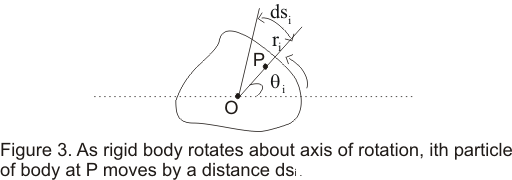# Angular Velocity Definition

## Angular velocity

• Consider a rigid body of arbitrary shape rotating about a fixed axis through point O and perpendicular to the plane of the paper as shown below in the figure-1• while the body is rotating each and every point in the body moves in a circle with their center lying on the axis of rotation and every point moves through the same angle during a particular interval of time
• Consider the position of a particle say ith particle at point P at a distance ri from point O and at an angle θi which OP makes with some reference line fixed in space say OX as shown below in the figure• If particle moves an small distance dsi along the arc of the circle in small amount of time dt then
dsi=vidt ----(1)
where vi is the speed of the particle
• dθ is the angle subtended by an arc of length dsi on the circumference of a circle of radius ri,so dθ( in radians) would be equal to the length of the arc divided by the radius
i.e.
dθ=dsi/ri =vidt/ri ----(2)
• distance dsi would vary from particle to particle but angle dθ swept out in a given time remain same for all the particles i.e. if particle at point P moves through complete circle such that
Then all the other particles of the rigid body moves through the angular displacement dθ=2π

• So rate of change of angle w.r.t time i.e. dθ/dt is same for all particles of the rigid body and dθ/dt is known as angular velocity ω of the rigid body so
ω=dθ/dt ----(3)
• Putting equation (3) in equation (2) we find
vi=ri(dθ/dt) =riω ---(4)
This shows that velocity of ith particle of the rigid body is related to its radius and the angular velocity of the rigid body
• Angular velocity of a rotating rigid body can either be positive or negative. It is positive when the body is rotating in anticlockwise direction and negative when the body id rotating in clockwise direction
• Unit of angular velocity radian per second (rad-s-1) and since radian is dimensionless unit of angle so dimension of angular velocity is [T-1]
• Instead of radians angles are often expressed in degrees. So angular velocity can also be expressed in terms of degree per second and degree per minute
• we know that
or π radians/1800=1 And this relation can be used for expressing angular velocity in degree to that of angular velocity in terms of radian